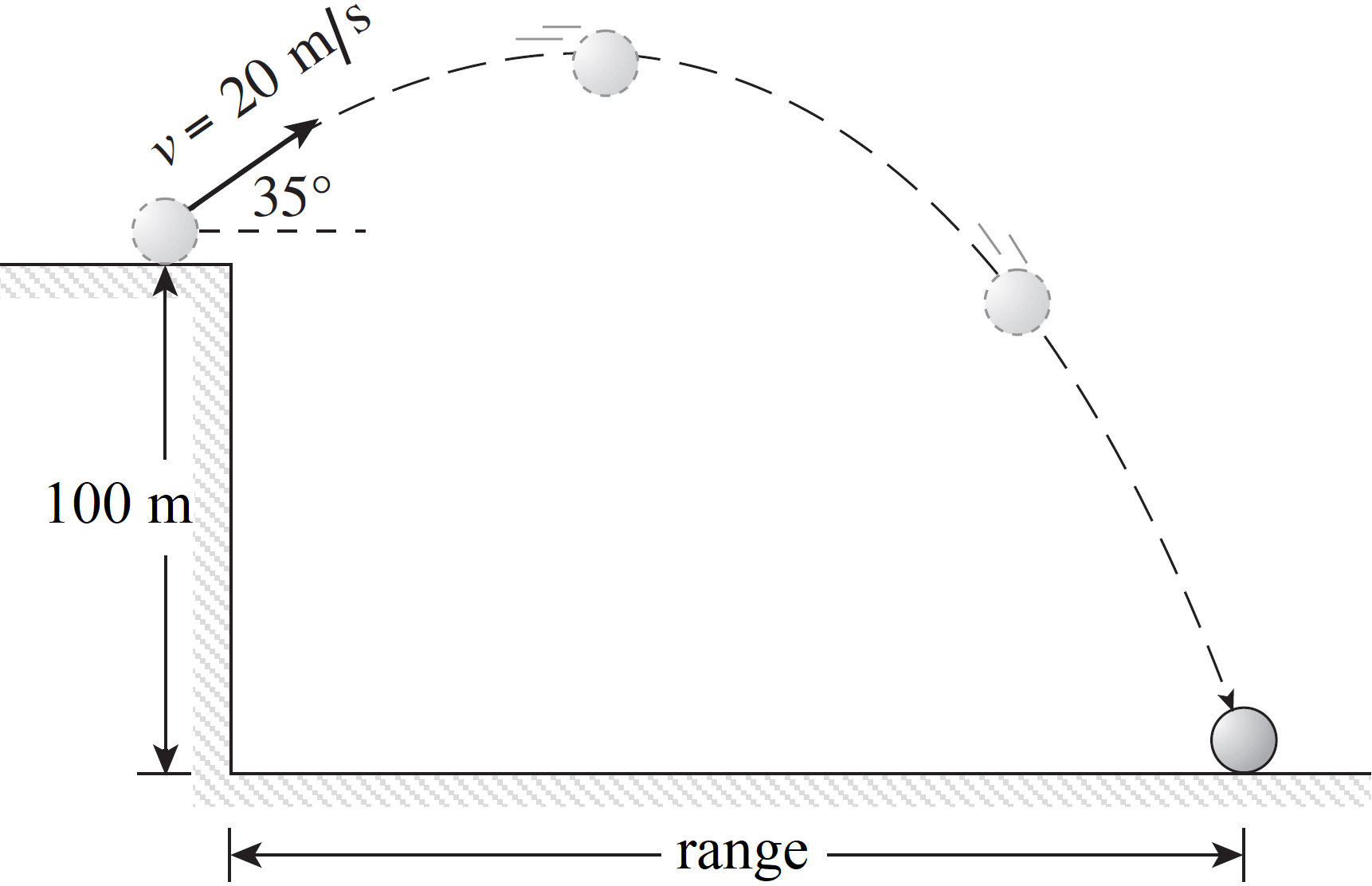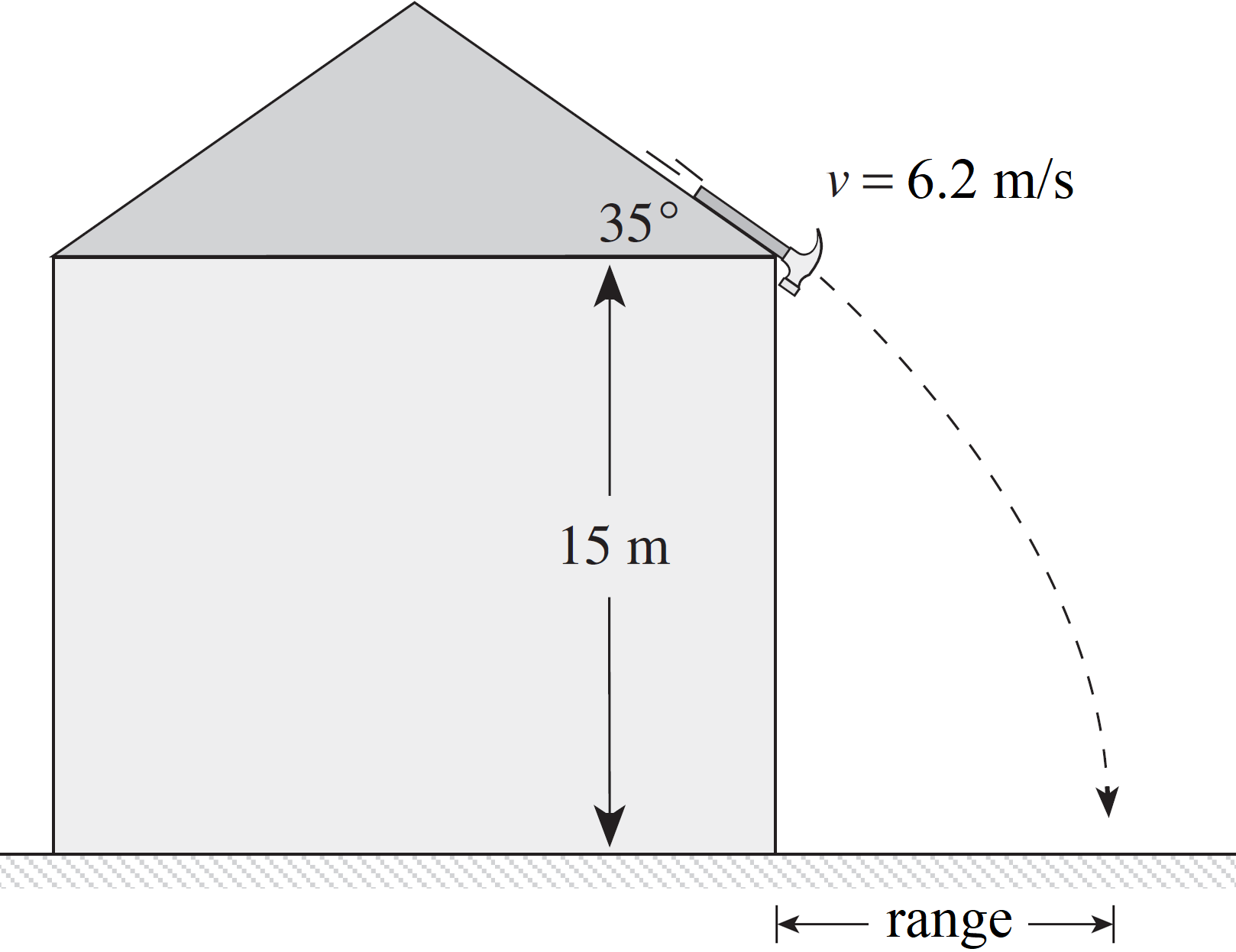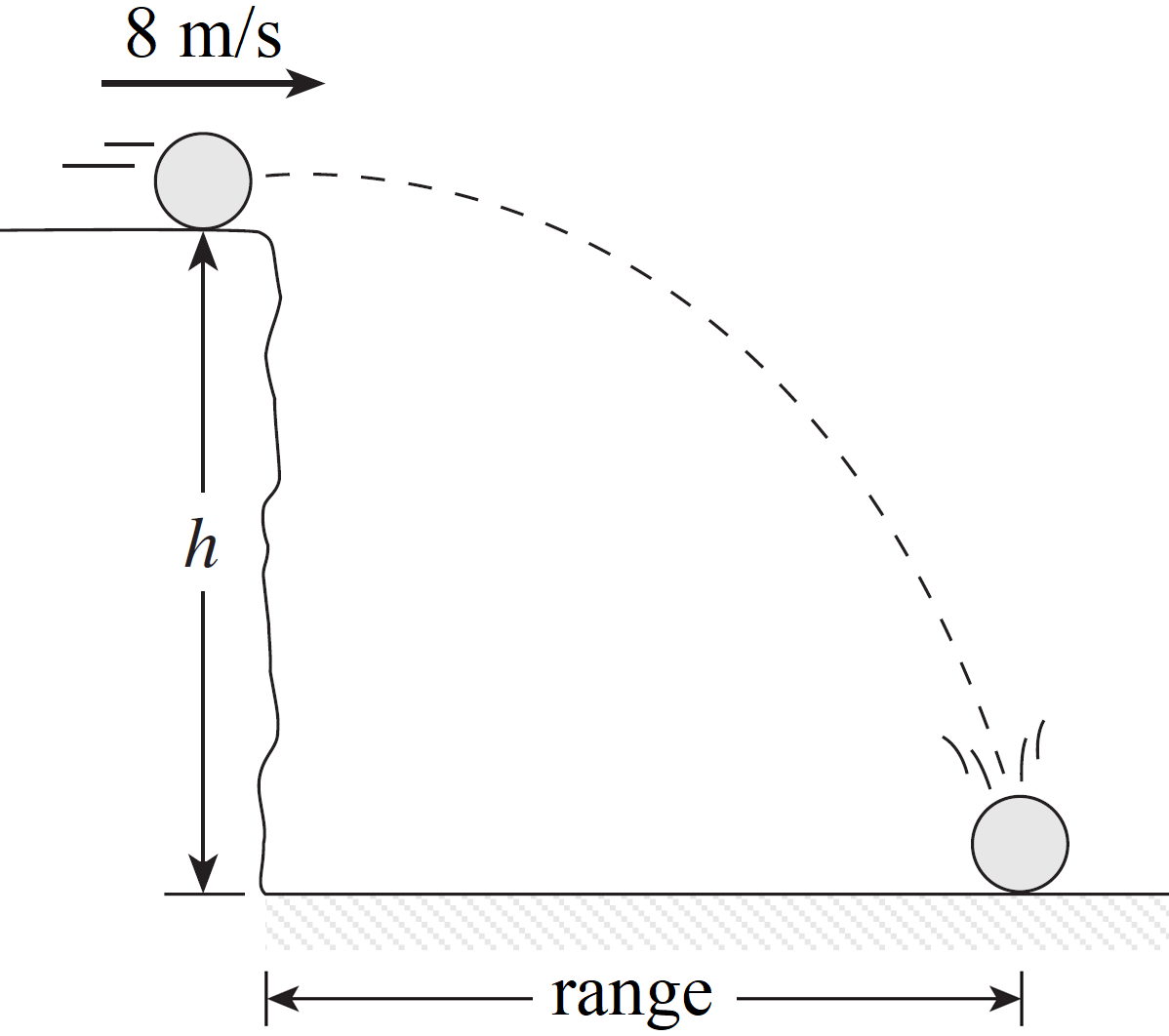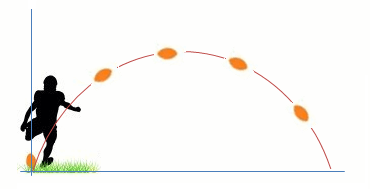# Two dimensional kinematics and projectile motion

### Two dimensional kinematics and projectile motion

#### Lessons

Notes:

Shortcut: Level Range Formulas for projectile returning to its original height:
• horizontal range: $d_x = \frac{v_i^2 \cdot sin2\theta}{g}$
• time of flight: $t=\frac{2v_i \cdot sin\theta }{g}$
• 1.
Type 1 – Projectile at an Upward Angle
A projectile is launched from the edge of a cliff 100 m above ground level with an initial speed of 20 m/s at an angle of 35° above the horizontal. Find:a)
the horizontal and vertical components of the launch speed.

b)
the velocity vector at the maximum height.

c)
the maximum height above the cliff top reached by the projectile.

d)
the time taken to reach the maximum height.

e)
the projectile's time of flight.

f)
the projectile's range.

g)
the projectile's impact velocity (magnitude and direction) with the ground.

• 2.
Type 2 – Projectile at a Downward Angle
A hammer slides down a roof sloped at 35° reaching a speed of 6.2 m/s before falling off.a)
How much time does it take to fall the 15 m to the ground?

b)
Determine the range as measured from the base of the house.

c)
Find the hammer's impact velocity (magnitude and direction) with the ground.

• 3.
Type 3 – Horizontally Launched Projectile
A ball rolls off a cliff at 8 m/s and hits the ground with a speed of 25 m/s.a)
Find the ball's impact velocity (magnitude and direction).

b)
How high (h) is the cliff?

c)
Determine the total time in the air.

d)
What is the range as measured from the base of the cliff?

• 4.
Projectile Returning to the Same Height – Level Range Formulas
Prove: Level Range Formulas
• time of flight: $t=\frac{2v_i \cdot sin\theta }{g}$
• horizontal range: $d_x = \frac{v_i^2 \cdot sin2\theta}{g}$

• 5.
Projectile Returning to the Same Height – Level Range Formulas
A football is kicked at an angle 65° with a velocity of 18 m/s. Determine the football's range and time of flight:a)
by using "Level Range Formulas".

b)
by using kinematic equations.